## Info

Publication number
JP2010223649A
JP2010223649A JP2009069378A JP2009069378A JP2010223649A JP 2010223649 A JP2010223649 A JP 2010223649A JP 2009069378 A JP2009069378 A JP 2009069378A JP 2009069378 A JP2009069378 A JP 2009069378A JP 2010223649 A JP2010223649 A JP 2010223649A
Authority
JP
Japan
Prior art keywords
signal
input
sum
difference
Prior art date
Legal status (The legal status is an assumption and is not a legal conclusion. Google has not performed a legal analysis and makes no representation as to the accuracy of the status listed.)
Pending
Application number
JP2009069378A
Other languages
Japanese (ja)
Inventor
Yasushi Aoyanagi
Hiroyuki Itohara

Original Assignee
Furukawa Automotive Systems Inc
Furukawa Electric Co Ltd:The

Priority date (The priority date is an assumption and is not a legal conclusion. Google has not performed a legal analysis and makes no representation as to the accuracy of the date listed.)
Filing date
Publication date
Application filed by Furukawa Automotive Systems Inc, Furukawa Electric Co Ltd:The, 古河Ａｓ株式会社, 古河電気工業株式会社 filed Critical Furukawa Automotive Systems Inc
Priority to JP2009069378A priority Critical patent/JP2010223649A/en
Publication of JP2010223649A publication Critical patent/JP2010223649A/en
Application status is Pending legal-status Critical

## Images

•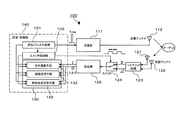•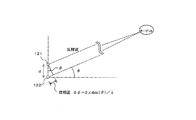•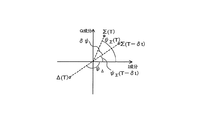•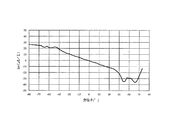•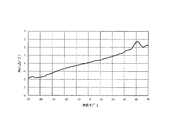•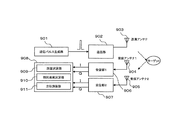## Abstract

A radar apparatus capable of measuring a phase with high accuracy by a phase monopulse method by simplifying a configuration of a receiving unit.
A radar apparatus 100 includes two receiving antennas 121 and 122 as a receiving system, a sum signal obtained by adding two receiving signals from the receiving antennas 121 and 122, and a difference signal that is a difference between the two signals. A hybrid circuit 123 that outputs a sum signal and a difference signal from the hybrid circuit 123, and an I signal and a Q signal whose phases are orthogonal to each other by IQ demodulation of the sum signal or difference signal input from the switch 124. Is received. The reception signals from the two reception antennas 121 and 122 are configured to be processed by the reception unit 125 of one series by switching with the switch 124.
[Selection] Figure 1

## Description

The present invention relates to a radar apparatus that detects a target and detects its direction by a phase monopulse method.

Conventionally, in a radar apparatus with limited dimensions, it is common to use a phase monopulse method to detect the azimuth (angle) of a target. As shown in FIG. 2, when a reflected wave reflected by one target is received by two receiving antennas, a phase difference formed by the orientation of the target is generated between the two receiving antennas. The orientation of the target is measured using the phase difference.

A configuration example of a conventional radar apparatus that performs angle measurement using the phase monopulse method is shown in FIG. The pulse signal generated by the transmission pulse generation unit 901 is up-converted to a predetermined frequency by the transmission unit 902 and output to the transmission antenna 903, and the transmission wave is radiated from the transmission antenna 903 into the air. The radiated transmission wave is reflected by the target and received by the two receiving antennas 904 and 905. The reception signals received by the reception antennas 904 and 905 are input to the reception units 906 and 907 and down-converted. Is output. The calculation unit 908 is provided with a distance calculation unit 909, a relative speed calculation unit 910, and an azimuth calculation unit 911, and each calculates a distance to the target, a relative speed, and an azimuth.

Patent Document 1 describes a phase monopulse radar including a plurality of reception antennas and the same number of reception units. The radar described in Patent Document 1 further includes a camera device, which can be used to specify the azimuth angle region where the target exists. This prevents erroneous detection of the orientation of the target due to phase folding.

Patent Document 2 describes a method for accurately estimating the arrival direction of a broadband signal. Here, two antennas are used, and signals received by the respective antennas are input to the hybrid circuit to create a sum signal (Σ) and a difference signal (Δ). The sum signal (Σ) and the difference signal (Δ) output from the hybrid circuit are input to the receiving unit, and the arrival direction of the wideband signal is estimated using the output from the receiving unit. When the frequency band of the signal is divided and the directions in which | Δ / Σ | falls (minimum) in each divided band coincide with each other, it is estimated that the true front direction (azimuth 0 °) at that time is the signal arrival direction is doing. This method is also one of the phase monopulse methods.

JP 2007-263986 A JP 2005-156521 A

In the phase monopulse radar device, two or more receiving antennas for receiving reflected waves are provided, and at least two receiving units are provided to perform phase monopulse processing using the received signals received by the receiving antennas. ing. The receiving unit for each system is provided with an amplifier that amplifies the received signal, a device for down-conversion, a device that outputs an I signal and a Q signal using an IQ demodulation method, and the like. For this reason, when a plurality of such receiving systems are provided, there is a problem that the size of the receiving unit increases and the cost also increases.

In addition, since signals received by two or more receiving antennas are processed by different receiving units and output to the calculation unit, the reception processing time required to reach the calculation unit after being received by the receiving antenna is received. If they differ from one part to another, there is a possibility that the arithmetic part cannot correctly perform synchronization. For this reason, it is necessary to manage the line length and the like in the receiving unit with high accuracy so that the reception processing times are almost the same regardless of which receiving unit is processed, which also causes an increase in cost.

The present invention has been made in view of the above problems, and an object of the present invention is to provide a radar apparatus capable of measuring a phase with high accuracy by a phase monopulse method with a simple configuration of a receiving unit.

In the present invention having the above configuration, two or more receiving antennas can be connected to one receiving unit via a switch, thereby simplifying the configuration of the receiving unit and performing phase measurement with high accuracy by the phase monopulse method. It becomes possible.

According to another aspect of the radar apparatus of the present invention, the switch connects the first input terminal and the second input terminal to the output terminal alternately, so that the sum signal and the difference signal are connected. It outputs alternately. By alternately outputting the sum signal and the difference signal to the receiving unit to perform reception processing, a temporal shift between the sum signal and the difference signal can be reduced.

Another aspect of the radar apparatus of the present invention is characterized in that the switch periodically performs switching between the first input terminal and the second input terminal at a predetermined time interval δt. By performing switch switching at a constant period, it becomes easy to synchronize with the radiation of the transmission wave from the transmission antenna.

Another aspect of the radar apparatus of the present invention includes a switch control unit that outputs the selection signal to the switch when the pulse signal is input from the transmission pulse generation unit. Thereby, switching of the switch can be performed in synchronization with the output of the pulse signal from the transmission pulse generator.

In another aspect of the radar apparatus of the present invention, the arithmetic unit is configured to input the sum signal or the difference signal I signal and the Q signal input from the receiver unit, and the difference signal or the sum signal input last time from the receiver unit. The azimuth is calculated using the corrected I signal and Q signal obtained by correcting the amount of change that changes during the time interval δt with respect to the I signal and Q signal of the signal. Thereby, the sum signal and difference signal used for calculation of the ratio can be made at the same time.

In another aspect of the radar apparatus of the present invention, the corrected I signal and Q signal are the difference signal or the sum signal I signal previously inputted with a phase change during the time interval δt due to the Doppler shift. And the Q signal is corrected and calculated. The change in the difference signal or the sum signal during the time interval δt can be corrected using the phase change due to the Doppler shift.

In another aspect of the radar apparatus of the present invention, the calculation unit calculates an imaginary part of a ratio between the sum signal and the difference signal to calculate the azimuth of the target. Depending on the characteristics of the receiving antenna, the azimuth can be calculated using the imaginary part of the ratio between the sum signal and the difference signal.

Another aspect of the radar apparatus of the present invention is characterized in that the calculation unit calculates a real part of a ratio between the sum signal and the difference signal to calculate the azimuth of the target. Depending on the characteristics of the receiving antenna, the azimuth can be calculated using the real part of the ratio of the sum signal and the difference signal.

According to the present invention, there is provided a radar device capable of measuring a phase with high accuracy by a phase monopulse method by connecting two or more receiving antennas to a single receiving unit through a switch and simplifying the configuration of the receiving unit. It becomes possible to provide.

It is a block diagram which shows the structure of the radar apparatus which concerns on embodiment of this invention. It is explanatory drawing for demonstrating the measuring method of the direction by a phase monopulse system. It is explanatory drawing which represented the sum signal and the difference signal on the complex plane. It is a graph which shows the change of the imaginary part of ratio of a sum signal and a difference signal with respect to an azimuth | direction. It is a graph which shows the change of the real part of ratio of a sum signal and a difference signal with respect to an azimuth | direction. It is a block diagram which shows the structure of the conventional radar apparatus which measures an azimuth | direction using a phase monopulse system.

A radar apparatus according to a preferred embodiment of the present invention will be described in detail with reference to the drawings. Each component having the same function is denoted by the same reference numeral for simplification of illustration and description.

The configuration of the radar apparatus according to the first embodiment of the present invention will be described with reference to FIG. FIG. 1 is a block diagram illustrating a configuration of a radar apparatus 100 according to the present embodiment. The radar apparatus 100 according to the present embodiment, as a transmission system, outputs a high-frequency pulse signal by up-converting a transmission pulse generation unit 101 that generates a pulse signal and a pulse signal input from the transmission pulse generation unit 101 into a predetermined frequency band. And a transmission antenna 112 that receives a high-frequency pulse signal from the transmission unit 111 and radiates a transmission wave. As a transmission system of the radar apparatus 100, for example, a transmission baseband pulse signal having a pulse width of about 1 ns is emitted from the transmission pulse generation unit 101, and this is upconverted to about 26.5 GHz by the transmission unit 111 and radiated from the transmission antenna 112. It can be configured to be.

In addition, as a receiving system, two reception antennas 121 and 122 that receive a reflected wave returned from a transmission wave radiated from the transmission antenna 112 and two reception signals from the reception antennas 121 and 122 are input. A hybrid circuit 123 that outputs a sum signal (Σ) obtained by adding the two and a difference signal (Δ) that is the difference between the two, a first input terminal 124a that inputs the sum signal from the hybrid circuit 123, and a difference signal. A switch 124 having a second input terminal 124b for output and an output terminal 124c for selecting one of the sum signal and the difference signal and outputting the result. The sum signal or the difference signal input from the switch 124 is IQ-demodulated to each other. Receiving unit 125 that outputs an I signal and a Q signal whose phases are orthogonal to each other, and a relative speed and direction of the target by inputting the I signal and the Q signal from the receiving unit 125 And a calculation unit 130 that calculates

The radar apparatus 100 is configured to be able to measure the azimuth of the target using a phase monopulse method. That is, the transmission system is configured by one system and includes one transmission antenna 112, whereas the reception system includes two reception antennas 121 and 122 that are arranged apart by a predetermined distance d. Two reception signals received and output by the reception antennas 121 and 122 are input to the hybrid circuit 123, converted into two signals of a sum signal and a difference signal, and output. As described above, the radar apparatus 100 according to the present embodiment is also configured to output two reception signals (sum signal and difference signal) using the two reception antennas 121 and 122. However, only one system is provided for the receiving unit 125 with complicated management of the line length and the like. Thereby, the radar device 100 can be easily reduced in size and price.

The radar apparatus 100 is configured to include a switch 124 in order to process the two reception signals required in the phase monopulse method by one system receiving unit 125, and the switch 124 alternately switches the two reception signals. The received signal selected here is output to the receiving unit 125 for signal processing. The switch 124 is connected to the output side of the hybrid circuit 123 that outputs two reception signals of the sum signal and the difference signal, and the switch 124 selects one of the two reception signals of the sum signal and the difference signal. This is output to the receiving unit 125 connected to the output terminal 124c.

In order to alternately select one of the sum signal and the difference signal by the switch 124, the switch control unit 102 is provided to control the switch 124. The switch control unit 102 receives a pulse signal from the transmission pulse generation unit 101, and using this as a trigger, the input ends 124a and 124b of the switch 124 are alternately switched and connected to the output end 124c. By using the pulse signal output from the transmission pulse generation unit 101 as a trigger to switch the switch 124, the reception signal that has received the reflected wave with respect to the transmission wave radiated from the transmission antenna 112 is received by the reception unit 125. Can be processed reliably.

Using the I signal and the Q signal output from the reception unit 125 to the calculation unit 130, the calculation unit 130 calculates target position information. In the radar apparatus 100, the calculation unit 130 includes the azimuth calculation unit 131 in order to measure the azimuth of the target using the phase monopulse method. The calculation unit 130 includes a distance calculation unit 132 for calculating the distance to the target and a relative speed calculation unit 133 for calculating the relative speed of the target. The distance, target orientation, and target relative speed can be provided.

In the radar apparatus 100 of the present embodiment, the calculation unit 130 including the azimuth calculation unit 131, the distance calculation unit 132, and the relative speed calculation unit 133 is provided in the calculation / control unit 140 that is a digital calculation device. In addition, a transmission pulse generation unit 101 that transmits a pulse signal to the transmission unit 111 and the switch control unit 102 and a switch control unit 102 that controls the switch 124 are also provided in the calculation / control unit 140.

In the radar apparatus 100 according to the present embodiment, the sum signal and the difference signal of the received signals are switched by the switch 124 and are alternately processed by the receiving unit 125. Therefore, the I signal of the sum signal input to the arithmetic unit 130, There is a time lag δt between the Q signal and the I signal and Q signal of the difference signal. This time lag δt is equal to the switching period of the switch 124, that is, the period in which the pulse signal is output from the transmission pulse generator 101. When the radar apparatus 100 and the target are stationary, even if there is such a time lag, the position information of the target does not change and there is no problem.

However, when at least one of the radar device 100 and the target is moving, the distance and direction to the target change during this time lag δt. The distance to the target can be calculated using only the latest signal of either the sum signal or the difference signal. On the other hand, since it is necessary to use both the sum signal and the difference signal for the calculation of the azimuth, the measurement accuracy of the azimuth may be lowered depending on the time lag δt and the magnitude of the relative speed.

Therefore, in the radar apparatus 100 according to the present embodiment, the azimuth of the target is calculated by correcting the deviation between the sum signal and the difference signal due to the time lag δt as described above, whereby the time lag δt and the relative speed are calculated. Even if is somewhat large, the orientation of the target can be calculated with high accuracy. When at least one of the radar apparatus 100 and the target is moving, a frequency shift due to the Doppler effect occurs in the received signal. The relative speed calculation means 133 of the calculation unit 130 performs FFT (Fast Fourier Transform) processing on the I signal and Q signal input from the reception unit 125 to calculate the frequency shift fd due to the Doppler effect, and calculates the relative speed therefrom. is doing. In the azimuth calculating means 131, the azimuth of the target is corrected using the frequency shift fd calculated by the relative speed calculating means 133. In the radar apparatus 100 of this embodiment, the time lag δt can be set to about 10 ms. Further, the frequency shift fd due to the Doppler effect is a low frequency (long cycle) within ± 6 kHz.

Hereinafter, a correction method for azimuth calculation performed by the radar apparatus 100 of the present embodiment will be described. Here, it is assumed that the azimuth of the target is calculated using the sum signal measured at time T−δt and the difference signal measured at time T after δt. In this case, it is necessary to correct the sum signal by estimating and changing the time lag δt. The azimuth may be calculated using the difference signal measured at time T−δt and the sum signal measured at time T after δt, and in that case, the change between the time lag δt with respect to the difference signal Is estimated and corrected. Thereby, it is possible to calculate the orientation of the target using the sum signal and the difference signal at the same time.

When two receiving antennas 121 and 122 arranged at a distance d apart receive a reflected wave reflected by a target in the direction of azimuth θ, between the two radio waves received by the two receiving antennas 121 and 122 The phase difference φ is given by the following equation as shown in FIG.
Here, λ is the wavelength of the transmission wave.

The sum signal Σ (t) and the difference signal Δ (t) at time t can be expressed in complex form using the I and Q signals input from the receiving unit 125 to the computing unit 130 as follows.
The calculation unit 130 inputs I Σ (T-δt) and Q Σ (T-δt) at time T-δt and I Δ (T) and Q Δ (T) at time T from the reception unit 125. Thus, the arithmetic unit 130 has a sum signal Σ (T−δt) and a difference signal Δ (T). The relationship between the sum signal Σ (T−δt) and the difference signal Δ (T) is shown in FIG. FIG. 3 shows an example of the sum signal Σ (T−δt) and the difference signal Δ (T) on the complex plane. The figure also shows the relationship with the sum signal Σ (T) at time T. It shows.

As shown in FIG. 3, at time T, the phase ψ Σ (T) of the sum signal Σ (T) is equal to that of the sum signal Σ (T−δt) at time T−δt that the arithmetic unit 130 has. It is advanced by δψ from the phase ψ Σ (T−δt). Therefore, in order to calculate the azimuth with high accuracy, the calculation unit 130 calculates the phase change δψ of the sum signal Σ that progresses during the time lag δt, and corrects the sum signal Σ (T−δt) using this. The sum signal Σ (T) at time T is calculated. The phase change δψ can be calculated by the following equation.
Here, td (= 1 / fd) is a Doppler period, and fd is a Doppler frequency.

The corrected sum signal Σ (T) = I Σ (T) + jQ Σ (T) at time T is the sum signal Σ (T−δt) = I Σ (T−δt) + jQ measured at time T−δt. It can be obtained by advancing the phase by δψ with respect to Σ (T−δt), and can be calculated as follows using a rotation matrix.

A sum signal Σ (T) at time T is calculated by correcting the sum signal Σ (T−δt) at time T−δt, which the arithmetic unit 130 has, using the above equation. The azimuth can be calculated from the input difference signal Δ (T) at time T. That is, the imaginary part Im (Δ (T) / Σ (T)) of Δ (T) / Σ (T) is obtained, and the relationship between the azimuth θ and the imaginary part Im (Δ / Σ) is stored in a table format or the like in advance. By referring to what is being performed, the azimuth θ of the target at time T can be calculated.

An example of the change of the imaginary part of the ratio (Δ / Σ) of the sum signal and the difference signal with respect to the azimuth θ is shown in FIG. The relationship between the azimuth θ and the imaginary part of (Δ / Σ) as shown in FIG. 4 is measured in advance and stored in a memory or the like in the calculation unit 130 in a table format or the like. The azimuth calculating means 131 reads a table or the like representing the relationship between the azimuth θ and the imaginary part of (Δ / Σ) from a memory or the like, and can use this to calculate the azimuth θ of the target as described above. .

In the above description, the method of calculating the azimuth using the relationship between the azimuth θ and the imaginary part of (Δ / Σ) has been described. However, depending on the characteristics of the receiving antennas 121 and 122, the azimuth θ and (Δ / Σ) In some cases, it is easier to detect the direction using the relationship with the real part. In that case, the relationship between the azimuth θ and the real part of (Δ / Σ) is measured in advance, and is stored in a memory or the like in the calculation unit 130 in a table format or the like. The azimuth calculating means 131 also calculates the real part of the ratio between the two after using the sum signal and the difference signal input from the receiving section 125 and correcting as described above. The azimuth θ can be calculated by referring to a table or the like stored in the memory or the like for the real part. An example of the relationship between the azimuth θ and the real part of (Δ / Σ) is shown in FIG.

As described with reference to the above embodiment, according to the radar apparatus of the present invention, it is possible to measure the azimuth by the phase monopulse method using one system of receiving unit, thereby reducing the size and price of the radar apparatus. It becomes possible to plan. In addition, it is possible to provide a radar device that is easy to handle because there is no need to manage the line length between the receiving units, which is a problem when there are two or more receiving units.

The description in the present embodiment shows an example of the radar apparatus according to the present invention, and the present invention is not limited to this. The detailed configuration and detailed operation of the radar apparatus according to the present embodiment can be changed as appropriate without departing from the spirit of the present invention.

DESCRIPTION OF SYMBOLS 100 Radar apparatus 101 Transmission pulse generation part 102 Switch control part 111 Transmission part 112 Transmission antenna 121,122 Reception antenna 123 Hybrid circuit 124 Switch 124a, 124b Input terminal 124c Output terminal 125 Reception part 130 Calculation part 131 Direction calculation means 132 Distance calculation means 133 Relative Speed Calculation Means 140 Calculation / Control Unit

## Claims (8)

1. A transmission pulse generator for generating a pulse signal;
A transmitter that up-converts the pulse signal input from the transmission pulse generator to a predetermined frequency band and outputs a high-frequency pulse signal;
One transmission antenna that radiates a transmission wave by inputting the high-frequency pulse signal from the transmission unit,
Two or more receiving antennas that receive a reflected wave returned from the target reflected by the transmission wave radiated from the transmitting antenna and output two received signals;
A hybrid circuit that outputs a sum signal obtained by adding the two received signals input from the two or more receiving antennas and a difference signal obtained by subtracting the other from one of the two received signals;
Connected to the first input terminal for inputting the sum signal from the hybrid circuit, the second input terminal for inputting the difference signal, and the first input terminal or the second input terminal according to a predetermined selection signal And a switch having an output terminal for outputting the sum signal or the difference signal,
A receiver that IQ-demodulates the sum signal or difference signal input from the switch and outputs an I signal and a Q signal whose phases are orthogonal to each other;
The I signal and the Q signal are input from the receiver, and the relative speed of the target is calculated from the frequency shift caused by the Doppler shift of the I signal and the Q signal, and the ratio of the sum signal and the difference signal is calculated. And a calculation unit for calculating the azimuth of the target.
2. The switch outputs the sum signal and the difference signal alternately by alternately connecting the first input terminal and the second input terminal to the output terminal. The radar apparatus according to 1.
3. The radar apparatus according to claim 1, wherein the switch periodically switches between the first input terminal and the second input terminal at a predetermined time interval δt.
4. The radar apparatus according to claim 1, further comprising: a switch control unit that outputs the selection signal to the switch when the pulse signal is input from the transmission pulse generation unit.
5. The arithmetic unit is configured such that the I signal and the Q signal of the sum signal or the difference signal input from the receiving unit and the I signal and the Q signal of the difference signal or the sum signal input from the receiving unit last time are the time intervals. 5. The radar apparatus according to claim 3, wherein the azimuth is calculated using a corrected I signal and Q signal obtained by correcting a change amount that changes during δt.
6. The corrected I signal and Q signal are calculated by correcting the phase change during the time interval δt due to the Doppler shift with respect to the previously input difference signal or sum signal I signal and Q signal. The radar apparatus according to claim 5.
7. The radar apparatus according to claim 1, wherein the arithmetic unit calculates an imaginary part of a ratio between the sum signal and the difference signal to calculate the azimuth of the target.
8. The radar apparatus according to claim 1, wherein the calculation unit calculates a real part of a ratio between the sum signal and the difference signal to calculate the azimuth of the target.
JP2009069378A 2009-03-23 2009-03-23 Radar device Pending JP2010223649A (en)

## Priority Applications (1)

Application Number Priority Date Filing Date Title
JP2009069378A JP2010223649A (en) 2009-03-23 2009-03-23 Radar device

## Applications Claiming Priority (1)

Application Number Priority Date Filing Date Title
JP2009069378A JP2010223649A (en) 2009-03-23 2009-03-23 Radar device

## Publications (1)

Publication Number Publication Date
JP2010223649A true JP2010223649A (en) 2010-10-07

# Family

## Family Applications (1)

Application Number Title Priority Date Filing Date
JP2009069378A Pending JP2010223649A (en) 2009-03-23 2009-03-23 Radar device

## Country Status (1)

JP (1) JP2010223649A (en)

## Cited By (1)

* Cited by examiner, † Cited by third party
Publication number Priority date Publication date Assignee Title
EP3236278A1 (en) * 2016-04-20 2017-10-25 Rohde & Schwarz GmbH & Co. KG Directional antenna module

## Citations (2)

* Cited by examiner, † Cited by third party
Publication number Priority date Publication date Assignee Title
JPH11160423A (en) * 1997-11-28 1999-06-18 Toyota Motor Corp Radar
JP2007155411A (en) * 2005-12-01 2007-06-21 Tdk Corp Radar system and high-frequency module

## Patent Citations (2)

* Cited by examiner, † Cited by third party
Publication number Priority date Publication date Assignee Title
JPH11160423A (en) * 1997-11-28 1999-06-18 Toyota Motor Corp Radar
JP2007155411A (en) * 2005-12-01 2007-06-21 Tdk Corp Radar system and high-frequency module

## Cited By (1)

* Cited by examiner, † Cited by third party
Publication number Priority date Publication date Assignee Title
EP3236278A1 (en) * 2016-04-20 2017-10-25 Rohde & Schwarz GmbH & Co. KG Directional antenna module

## Similar Documents

Publication Publication Date Title
JP6304257B2 (en) Radar apparatus, vehicle, and moving body speed detection method
US9971028B2 (en) Method and apparatus for detecting target using radar
EP2741430B1 (en) Phased array transmission device
EP2983007A1 (en) Radar apparatus and object sensing method
JP5093298B2 (en) Direction detection device
WO2015190565A1 (en) Radar device using chirp wave, and method for signal processing in radar device
JP4769684B2 (en) Electronic scanning radar equipment
JP4232570B2 (en) Radar equipment for vehicles
DE112007003175B4 (en) Electronically scanning radar system
DE60025064T2 (en) Radar device using digital beamforming technology
JP5009282B2 (en) System and method for coherently combining multiple radars
US7429947B2 (en) Radar apparatus for detecting distance or velocity with respect to an object
DE10354872B4 (en) A device for detecting a direction of a target using a phase difference of radio wave signals received over a plurality of channels
US8797213B2 (en) Methods and systems for estimating angle of arrival

## Legal Events

Date Code Title Description
A621 Written request for application examination

Free format text: JAPANESE INTERMEDIATE CODE: A621

Effective date: 20120109

A977 Report on retrieval

Free format text: JAPANESE INTERMEDIATE CODE: A971007

Effective date: 20130123

A131 Notification of reasons for refusal

Free format text: JAPANESE INTERMEDIATE CODE: A131

Effective date: 20130405

A02 Decision of refusal

Free format text: JAPANESE INTERMEDIATE CODE: A02

Effective date: 20130726